## LetsPlayMaths.Com

WELCOME TO THE WORLD OF MATHEMATICS

# Class 5 Perimeter

Perimeter

Perimeter of a Triangle

Perimeter of a Rectangle

Perimeter of a Square

Perimeter Test

Perimeter Worksheet

## Perimeter

Perimeter is the sum of the lengths of the sides of any figure. Letís find out perimeter of triangle, rectangle, square and quadrilateral.

## Perimeter of a Triangle

Perimeter of a triangle can be find out by adding the length of itís three sides. Letís see some examples.

Example 1. Find out the perimeter of the below given triangle.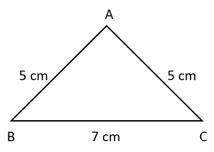Solution.Length of side AB = 5 cm
Length of side BC = 7 cm
Length of side AC = 5 cm
Perimeter of the triangle = Length of AB + Length of BC + Length of AC
= 5 cm + 7 cm + 5 cm
= 17 cm
So, the perimeter of the triangle is 17 cm.

Example 2. Find out the perimeter of the below given triangle.Solution.Length of side AB = 3 cm
Length of side BC = 4 cm
Length of side AC = 5 cm
Perimeter of the above triangle = Length of AB + Length of BC + Length of AC
= 3 cm + 4 cm + 5 cm
= 12 cm
So, the perimeter of the triangle is 12 cm.

## Perimeter of a Rectangle

Perimeter of a rectangle is sum of all four sides. We know that opposite sides of a rectangle are equal.Perimeter of a rectangle = 2 x (Length + Breadth)

Example 1. Find out the perimeter of a rectangle whose length is equal to 5 cm and breadth is equal to 3 cm.

Solution.
Perimeter of the rectangle = 2 x (Length + Breadth)
= 2 x (5 cm + 3 cm)
= 2 x 8 cm
= 16 cm
So, the perimeter of the rectangle is equal to 16 cm.

## Perimeter of a Square

Perimeter of a square is sum of all four sides. We know all the sides of a square are equal.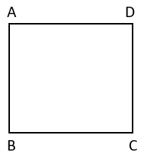Perimeter of a square = 4 x Side length

Example 1. The side of a square is 5 cm. Find the perimeter.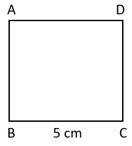Solution.Perimeter of the square = 4 x Side length = 4 x 5 cm = 20 cm.
So, the perimeter of the square is equal to 20 cm.

Perimeter of a quadrilateral is the sum of the length of all four sides.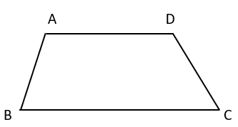Perimeter of the quadrilateral = Length of AB + Length of BC + Length of CD + Length of AD

Example 1. Find the perimeter of below given quadrilateral.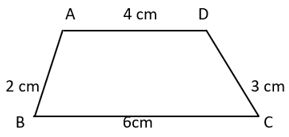Solution.Perimeter of the quadrilateral ABCD = 2 cm + 6 cm + 3 cm + 4 cm
= 15 cm
So, the perimeter of the quadrilateral ABCD is equal to 15 cm.

Example 2. Find the perimeter of below given figure.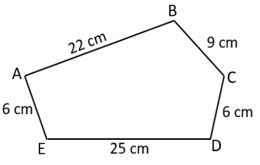Solution. Perimeter of the polygon = AB + BC + CD + DE + EA

= 22 cm + 9 cm + 6 cm + 25 cm + 6 cm

= 68 cm

So, the perimeter of the polygon is 68 cm.

Example 3. Find the perimeter of the below given figure.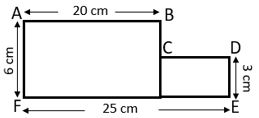Solution. In the above figure, AF = BE

=> AF = BC + DE

=> 6 cm = BC + 3 cm

=> BC = 3 cm

EF = AB + CD

=> 25 cm = 20 cm + CD

=> CD = 25 cm ñ 20 cm

=> CD = 5 cm

Perimeter of the above figure = AB + BC + CD + DE + EF + AF

= 20 cm + 3 cm + 5 cm + 3 cm + 25 cm + 6 cm

= 62 cm

Example 4. Perimeter of a rectangular field is 528 m. If length of the field is double the length of itís breadth then find out the length and breadth of the filed.

Solution. Perimeter of rectangle = 2 x ( Length + Breadth )    Length = A & Breadth = B

= 2 x (A + B)

Length of the rectangle is double the length of breadth.

A = 2B

=> 2 x (A + B) = Perimeter of rectangle

=> 2 x (2B + B) = 528 cm

=> 2 x (3B) = 528 cm

=> 6B = 528 cm

=> B = 528 ˜ 6

=> B = 88 cm

A = 2B

A = 2 x 88 cm = 176 cm

So, the length of the rectangle is 176 cm and breadth is 88 cm.

Example 5. Perimeter of a square field is 556 cm. What is the length of itís one side?

Solution. Perimeter of a square = 4 x A    (A = Length of each side)

4 x A = 556 cm

A = 556 ˜ 4 = 139 cm

So, length of each side of the square is 139 cm.

Example 6. Perimeter of a triangular field is 145 m. Length of two sides are 35 m and 45 m. What is the length of itís third side?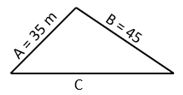Solution. Perimeter of a triangle = Total length of all the three sides

= A + B + C

35 m + 45 m + C = 145 m

=> C = 145 ñ (35 + 45)

=> C = 65 m

So, the length of the third side is 65 m.

Example 7. Find the cost of fencing a rectangular field of 70 m length and 50m width at the rate of Rs. 200 per meter.

Solution. Perimeter of the rectangular field = 2 x ( Length of the field + Breadth of the field)

= 2 x (70 + 50) = 2 x 120 = 240 m

Fence to be erected on 240 m at a rate of 200 per meter.

Cost of fencing = 240 m x Rs. 200 = Rs. 48000

So, cost of fencing is Rs. 48000

## Perimeter Test

Perimeter Test - 1

Perimeter Test - 2

## Class-5 Perimeter Worksheet

Perimeter Worksheet - 1

Perimeter Worksheet - 2

Perimeter Worksheet - 3